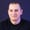FREELessons: 20Length: 1.2 hours• Overview
• Transcript

# 5.2 CSS Circles

In the previous lesson I showed you how to create triangles in CSS. Circles are just as easy to create and the technique is actually easier to understand.

## 7.Conclusion1 lesson, 01:47

### 5.2 CSS Circles

In the previous lesson, I showed you how to create triangles in CSS. Circles are just as easy to create, and the technique is actually easier to understand. So let's see an example. I have a document here with a div class circle, which is blank. And then an image of 200 by 200 with a class of round-image. So, let's see the circle first. Circle, we'll start with this background-color, so we can see it better. Let's do a green. And then set a width, let's say 10em, set a height, also 10em. And to make this round, all you gotta do is set a border-radius to half its width and height, so 5em. Save, and there you go, [LAUGH] that's a perfect circle. Now, I added this image because you can also create circles from images. So, round-image. We gotta set a width of 200 and a height of 200 pixels. And then a border-radius, oops, of 100 pixels. And now this image is also a circle. And there you go, really simple technique. Browser support is pretty good, it will work in all major browsers, except IE8.

Back to the top